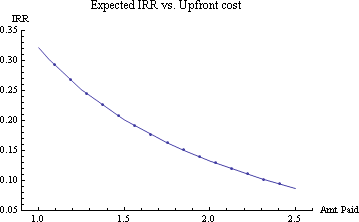# reversing the x-axis in a Mathematica graph

Sometimes you have data in which the dependent variable decreases as the independent variable increases, and for reasons of clarity in illustrating it, you want to flip the x-axis and show an increasing line (or vice versa). For example, sometimes you haveand you want to display it asUnfortunately, Mathematica’s default behavior is to show both axes growing as you go up and to the right, and there is no simple option to change that. You can achieve this however by transforming your data by hand so that it is displaying the desired way, and then setting the “Ticks” variable of the plotting function to display the Ticks in reverse order. You can’t just say “reverse,” you have to create a list of the new tick labels and where you want them to go. This is generally done with a call to the Table[] command.

I more commonly flip the X axis than the Y, and given data in {{x1,y1},{x2,y2} . . . {xn,yn}} format, the following code makes it very easy to do.

```xFlipper[listofXYpairs_] := Map[{Last[listofXYpairs][] + First[listofXYpairs][], 0} + {-1, 1}*# &, listofXYpairs]```

`Options[xFlippedTicks] = {numPoints -> 10, digits -> "All"};`

```xFlippedTicks[listofXYpairs_, OptionsPattern[]] := Table[{Last[listofXYpairs][] + First[listofXYpairs][] - i, If[OptionValue[digits] == "All", i, Round[i, 10^-OptionValue[digits] // N]]}, {i, First[listofXYpairs][], Last[listofXYpairs][], ( Last[listofXYpairs][] - First[listofXYpairs][])/ OptionValue[numPoints]-1}] ```

The numpoints variable sets how many points to show on the X axis. The digits variable can reduce the number of the digits shown in the tickmarks if needed to make the graph more useful or attractive.

The first graph above was produced with
``` ListPlot[returnPairs, PlotLabel -> "Expected IRR vs. Upfront cost", AxesLabel -> {"Amt Paid", "IRR"}, Joined -> True, Mesh -> True] ```

Given these two functions, we can transform it to the second graph above with
``` ListPlot[xFlipper[returnPairs], PlotLabel -> "Expected IRR vs. Upfront cost", Ticks -> {xFlippedTicks[returnPairs, numPoints -> 5], Automatic}, AxesLabel -> {"Amt Paid", "IRR"}, Joined -> True, Mesh -> True] ```

(The “automatic” in the Tick specification tells Mathematica to continue to handle the Y axis automatically.)